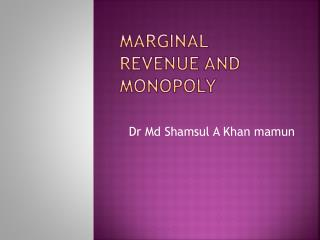# Marginal Revenue and Monopoly - PowerPoint PPT PresentationDownload PresentationMarginal Revenue and Monopoly

Marginal Revenue and Monopoly
Download Presentation## Marginal Revenue and Monopoly

- - - - - - - - - - - - - - - - - - - - - - - - - - - E N D - - - - - - - - - - - - - - - - - - - - - - - - - - -
##### Presentation Transcript

1. Marginal Revenue and Monopoly Dr MdShamsul A Khan mamun

2. What price and output charged and produced by a monopoly ? • To answer the question we need to compare cost of production and revenue; more specifically marginal cost and marginal revenue. • Demand curve of a monopolist firm is downward sloping to the right. In order to increase sales a monopolistic need to decrease price. Such reduction in price will

3. Continue.. • Continue to the point of unit elasticity of the demand curve. Because below the point reduction in sales price will not generate sufficient revenue to ensure profit maximization • Since a natural monopolistic desire to maximize profit, the maximum profit is attainable where its marginal revenue equals its marginal cost i.e MC=MR. That exactly will be the point of output and price of a monopolistic

4. Table: Firm’s profit maximization The table show that profit maximization condition is reached at the output level 5 with price level 120 Tk. Where MR=MC.

5. Graphical explanation At E, where MC intersect MR equilibrium of the maximum amount is found. Any move from E will loss some profit. Price is at G above E, and since P is above AC, the maximized profit is a positive profit. BLUE coloured zone.